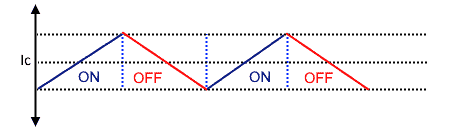# Minimum Capacitance Calculator

The minimum value for the capacitor is necessary to stabilize the input voltage due to the peak current requirement of a switching power supply.
Minimum value for the capacitor is necessary to stabilize the input voltage due to the peak current requirement of a switching power supply. This calculator calculates the Minimum Capacitance of a Step Down Converter.

### Result

• Minimum Capacitance
FMinimum Capacitance Voltage indicates

While pure DC current flows into the load, the specified capacitor will offer a conduit for AC ripples of inductor current. As a result, the capacitor will function as a filter.

Minimum Capacitance: The expressions for finding the value of the filter capacitor are derived from the relation ∆V = ∆Q/C, where Q is current × time.Minimum Capacitance

The capacitor is configured so that the maximum input voltage is equal to the standby capacitor voltage. Ideally, both are regarded equal, i.e. Vcmax = VDCmax, but in reality, the capacitor has ESR (Equivalent Series Resistance). Capacitor loss is influenced by the ESR factor. There are two ways to minimise the ESR factor for greater efficiency. By either paralleling capacitors or selecting capacitors with low ESR.

Minimum value for the capacitor is necessary to stabilize the input voltage due to the peak current requirement of a switching power supply.This calculator calculates the Minimum Capacitance of a Step Up Converter.

• Unitless

### Result

• Minimum Capacitance
FThe minimum value for the input capacitor is normally given in the data sheet. This minimum value is necessary to stabilize the input voltage due to the peak current requirement of a switching power supply. The best practice is to use low equivalent series resistance (ESR) ceramic capacitors. The dielectric material should be X5R or better. Otherwise, the capacitor can lose much of its capacitance due to DC bias or temperature

Best practice is to use low ESR capacitors to minimize the ripple on the output voltage. Ceramic capacitors are a good choice if the dielectric material is X5R or better. If the converter has external compensation, any capacitor value above the recommended minimum in the data sheet can be used, but the compensation has to be adjusted for the used output capacitance. With internally compensated converters, the recommended inductor and capacitor values should be used or the recommendations in the data sheet for adjusting the output capacitors to the application should be followed for the ratio of L × C.